### Home > CALC > Chapter 11 > Lesson 11.1.1 > Problem11-9

11-9.
1. Consider the infinite series below. For each, decide if it converges or diverges and justify your conclusion. State the test you used. Homework Help ✎

1.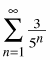2.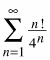3.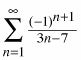4.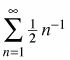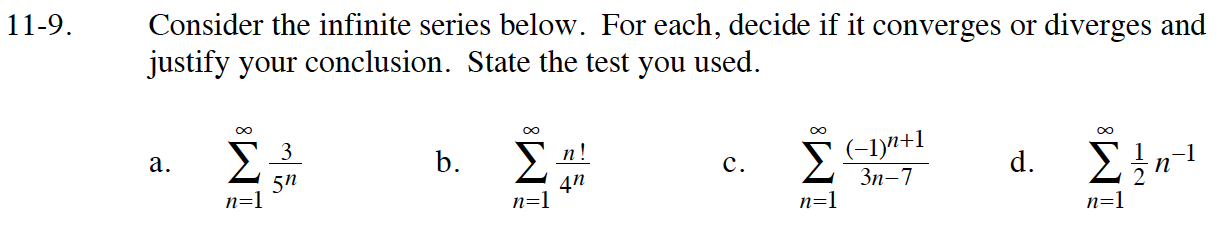This is a geometric series.

Use the Ratio Test.

This is an alternating series.

$=\frac{1}{2}\sum_{n=1}^\infty\frac{1}{n}$